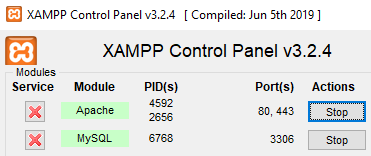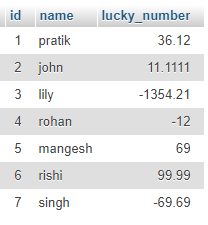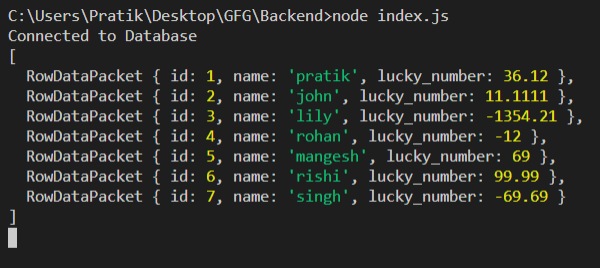GFG App
Open AppBrowser
Continue

# How to save connection result in a variable in Node.js ?

We are going to use the query function in MySQL library in node.js that will return our output as expected. Using this approach, we can save connection result in a variable in Node.js.

Setting up environment and Execution:

Step 1: Initialize node project using the following command.

`npm init`

Step 2: Turn on MySQL DatabaseStep 3: After creating the NodeJS application, Install the mysql module using the following command.

`npm install mysql`

Database Table: Our sample gfg_table database with look like this.Step 4: Create an index.js file with the following code.

## index.js

 `const mysql = require(``"mysql"``); ` ` `  `var` `db_con = mysql.createConnection({ ` `    ``host: ``"localhost"``, ` `    ``user: ``"root"``, ` `    ``password: ``""``, ` `    ``database: ``"gfg_db"` `}); ` ` `  `let output; ` ` `  `const setOutput = (rows) => { ` `    ``output = rows; ` `    ``console.log(output); ` `} ` ` `  `db_con.connect(async(err) => { ` `    ``if` `(err) { ` `        ``console.log(``"Database Connection Failed !!!"``, err); ` `        ``return``; ` `    ``} ` ` `  `    ``console.log(``"Connected to Database"``); ` ` `  `    ``let query = ``'SELECT * FROM users'``; ` `    ``db_con.query(query, (err, rows) => { ` `        ``if` `(err) { ` `            ``console.log(``"internal error"``, err); ` `            ``return``; ` `        ``} ` `         `  `        ``// This is the important function ` `        ``setOutput(rows); ` `    ``}); ` `}); `

Step 5: Run the index.js file using the following command.

`node index.js`

Output:My Personal Notes arrow_drop_up